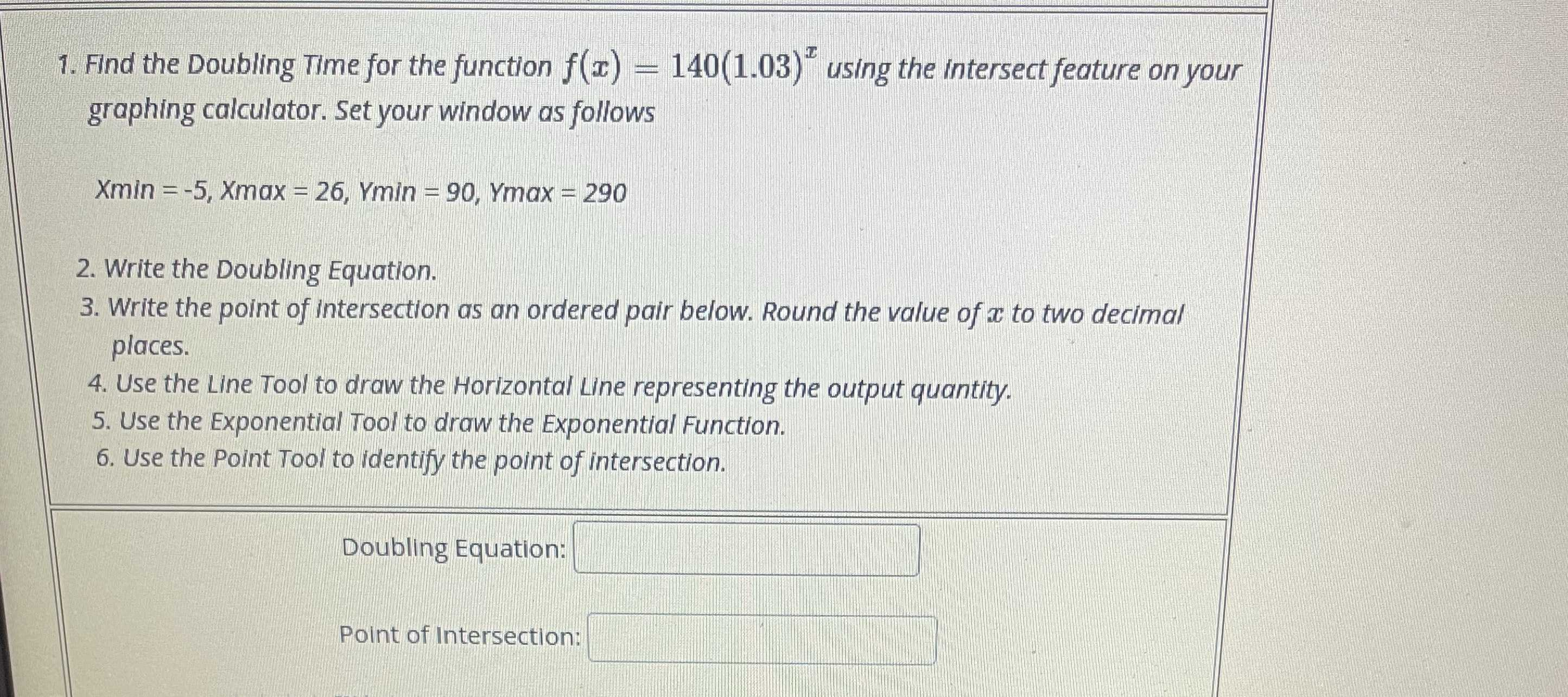### ¿Todavía tienes preguntas de matemáticas?

Pregunte a nuestros tutores expertos
Algebra
Pregunta1. Find the Doubling Time for the function $$f ( x ) = 140 ( 1.03 ) ^ { x }$$ using the intersect feature on your graphing calculator. Set your window as follows. $$X \min = - 5 , X \max = 26 , Ymin = 90 , Ymax = 290$$.

2. Write the Doubling Equation.

3. Write the point of Intersection as an ordered pair below. Round the value of $$x$$ to two decimal places.